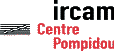Serveur © IRCAM - CENTRE POMPIDOU 1996-2005.Tous droits réservés pour tous pays. All rights reserved.

# State Space Sound Synthesis and a State Space Synthesiser Builder

Philippe Depalle, Stéphan Tassart

## Abstract

This paper deals with sound synthesis using state space models. It focuses on two realizations we made of state space synthesiser builders applied to the case of wind instruments and more generally to transmission lines networks. The first realization, written in Maple is devoted to symbolic manipulation such as the derivation of different formalisms - modal representation, ARMA filters or Kalman filtering equations. For efficiency purposes, a second version has been designed in C++ which directly reads the description of a physical instrument and computes its sound signal outputs.

## Introduction

We present recent developments of synthesis using State Space Models (SSM) [DM92] and a state space synthesiser builder applied to the case of wind instruments. SSM are particularly interesting since they attempt to unify two main categories of sound synthesis techniques: signal modeling and physical modeling. This approach relies on the state space formalism which is widely used in control system theory. It allows to take into account all the physical parameters of the system under study and benefits from the methods developed for automatic tracking of parameters values, such as adaptive filtering or Kalman filtering.

Physical models usually require the complete construction of a model for each new instrument. On the other hand our SSMs provide a methodology for modular construction of instruments [Tas94]. This construction is done automatically in our formal calculus environment. It allows to obtain different theoretical representations (state space equation, external or modal representation) and a synthesiser which simulates the instrument.

This paper focuses on the production of state space representation of hierarchical networks. Thus, we only briefly present the physical systems involved in wind instruments. We give some basics concerning the state space representation and we describe in detail the methodology that we used to combine modules together into networks of arbitrary complexity. In the third section, we apply this methodology to transmission lines, and in the last section we describe two software realizations of synthesiser builders.

## Physical Model of Tubes

The first class of physical systems we are interested in is that of wind instruments. We have to deal with acoustic tubes, junctions of two or more tubes and boundary conditions (such as reflection on the excitator and radiation in the air) [Smi92]. For each of this basic modules, we have to specify the degree of refinement of the physical description we intend to use.

### Acoustic Tubes

To model an acoustic tube, we use rather restrictive assumptions : we consider it a transmission line and we suppose its walls are perfectly rigid. These assumptions are standard for speech processing even though they are wrong especially for the vocal tract. We also take into account viscothermal losses [Mat94].

In order to obtain a discrete model, the acoustic tube is spatially discretized in N elementary tubes of equal length and given section. This spatial discretisation is directly related to the sampling period which is the exact delay for a round trip in an elementary tube.

For a lossless tube the usual description of propagating waves in terms of superposition of ingoing and outgoing waves leads to the Kelly-Lochbaum structure. This structure is made of two delays and is parameterized by one reflection coefficient which represents the discontinuity between two elementary tubes. For state space description,s we prefer the lattice structure which is obtained by putting all the delays in the upper or in the lower branch of the lattice and by normalizing input and output waves [Mat90]. In that way we represent an acoustic tube by a quadripole system with an N-dimensional state space internal vector. As we normalize input and output waves of the acoustic tube in the lattice representation, we have to denormalize the inputs and the outputs of the whole system to obtain physical values.

When taking into account viscothermal losses, we prove that the acoustic tube is still described by a generalization of the lattice filter. Following the delay we add a filter which approximates the frequency-dependent attenuation [Mat94]. The attenuation factor being proportional to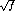(whereis the frequency), we use specific techniques to approximate it by a digital filter. We get the same structure for the state space equation than in the lossless case except for some scalars which are replaced by block matrices.

### Junctions of Acoustic Tubes

A wind instrument is an assembly of interconnected tubes. Connections of tubes are modeled by junction modules which implement Kirchoff's laws. At this time, we only consider two-port and three-port junctions. As in electrical circuit systems, networks have to be oriented. Consequently, we distinguish four three-port junctions.

### Boundary Conditions

The input boundary condition represents the loading of the excitator on the system and the output boundary condition, the radiation effect. A simple model for these boundary conditions is a frequency independent reflection coefficient. A more refined model consists of frequency dependent feedback loops which represent the load of the excitator or the radiation effect.

## State Space Sound Synthesis

### State Space Model

Most physical systems can be split up into basic components coupled according to a specific topology and characterized by physical parameters. The general expression of such a model without the restrictions of a linear system is eq. (1) where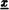,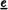and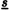are respectively the internal state vector, the input vector, and the output vector.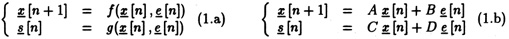Furthermore, a wide range of physical systems can be considered as linear dynamical systems. Then, the formal expression of such a model becomes (
1.b) where the system is now represented by four matrices (A, B, C, D) respectively the matrix of dynamics, the control matrix, the observation matrix and the direct link matrix [Kai80]. These matrices are directly derived from the topology and the parameters of the system under study. Then building the model of an instrument is equivalent to establishing its four representative matrices. Once the representation is obtained, the system can be simulated by driving it with input vectors. If evolving in time, the system is described by time dependent matrices.

### Systems Combination

In order to obtain the state space representation of systems composed of more elementary sub-systems, we developed a specific methodology. It consists of organizing the set of sub-systems into a hierarchical network. Then the SSR of each sub-systems is combined automatically with our formal calculus environment.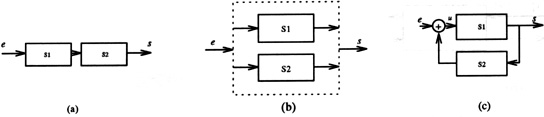Figure 1: Serial (a), parallel (b) and feedback (c) connections of two modules.
To build a network, we proceed by recursive combinations of two sub-systems. Considering two systems S1 and S2, they can be assembled (fig.
1) in a serial, parallel or feedback manner to create the system S. For these three combinations, the internal state space vector is= (1,2).

For the serial combination the output of S1 is connected to the input of S2 and we have=2 and=1. For the parallel combination, input and output are simply a concatenation := (1,2) and= (1,2). And for the feedback combination, we have=1 =2 and=1 -2.

Notice that these combinations have been defined in order to construct networks in a very general way. They do not directly have a physical meaning. In particular, the connection of two acoustic tubes is not a simple serial connection but is done through the connection of two quadripoles which involves 4 serial, 2 parallel and 1 feedback connections (fig. 3).

### From State Space to Other Representations

Formal description is very useful for performing modifications such as the manipulation of state space equations or for deriving other representations such as modal or external representations.

For example, in order to identify an unknown parameter by using the Kalman extended filtering techniques, we classically add this parameter as an extra state space variable to the internal state vector. Consequently, we have to derive automatically a new state space equation corresponding to the enlarged state vector.

Establishing an external representation of physical systems is very useful, for example, for performing real-time synthesis driven by physical parameters. The synthesis becomes very efficient computationally speaking. Such an external representation is obtained by evaluating :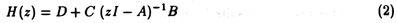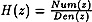is a transfer matrix and it reduces to a scalar expression when input and output vectors are one-dimensional. In this case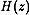is the transfer function corresponding to an ARMA filter.

Modal representation of physical systems is well adapted to the context of sound synthesis because of the structure of the sound output. Modal synthesisers such as Modalys (previously known as Mosaic) need a data base of modes adapted to the structure which is to be excited. Modes can be obtained by establishing the state space equation for the structure and by diagonalizing the matrix A of dynamics. This is performed by formal or numerical calculus.

### Global Representation of Linear Systems

In the linear case, a global representation ( A, B, C, D ) of a system network can be entirely deduced from the representation ( Ai, Bi, Ci, Di ) of each of the components. Serial, parallel and feedback connections are described in the following equations where+ and are- respectively set to (I - D1D2)-1, (I - D2D1)-1, with D1- =+D2.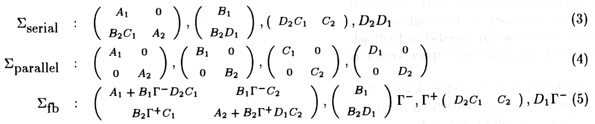### Modular Representation

The global approach for system representation is not efficient from a computational standpoint: simulating a 50 cm tube at 44.1kHz requires more than 6000 multiplication/additions per sample. To avoid this problem we develop a modular approach which simulates a whole system by propagating signal flows between modules. This approach is similar to the one developed in Music V, C-sound, Max, and waveguides.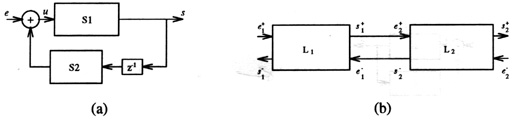Figure 2: (a) Simulable feedback; (b) serial q-junction of two quadripoles.

We are interested in knowing in advance whether a network is computationally simulable or not. (By simulable we mean simply: ``capable of being simulated''.) Let S be a module network, S is simulable if its state equations (i.e.and g) are explicit expressions of the component module equations. By extension, we will say that a module is simulable for a given type of combination if any combination of the given type results in a simulable network.

### Simulable Modules Networks

Serial and parallel connected modules are simulable provided that all components are themselves simulable. On the contrary, feedback (fig.
1.c) can not be simulated because[n] results from the following implicit equation[n] = g1([n] + g2([n])).

However, if the feedback loop is broken by at least one delay, as shown in figure 2.a, we get an explicit expression for the output ([n] = g1([n] + g2([n-1])))(see also [FC90]).

## Transmission Line Application

One-dimensional transmission lines or junctions are quadripoles, i.e. a particular kind of system with two distinct inputs (-,+), and outputs (+,-). These quadripoles may be connected together with specific serial q-junctions (fig. 2.b) and parallel q-junctions12. These q-junctions are described as a hierarchical network of parallel, serial and feedback connections (fig. 3 and 4).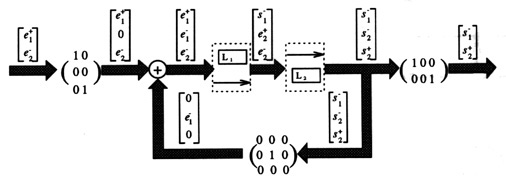Figure 3: decomposition of a serial q-junction.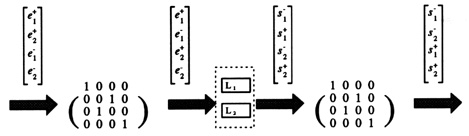Figure 4: decomposition of a parallel q-junction.

A serial q-junction between two quadripoles involves a feedback connection. It turns out that serial q-junctions of quadripoles can not be simulated in the general case, but can be simulated if each incriminated loop is broken by a delay.
Basically a delay can be extracted either in the upper line (the ingoing waves) or in the lower line (the outgoing waves) (fig.
5). We call them respectively quad-up and quad-down. We provide also an orientation to a quad so that at least one delay is nested on its left side (i.e. e+ input or s- output ).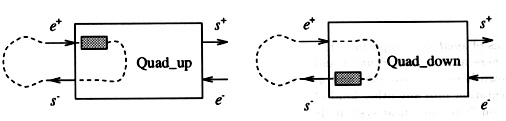We deduce some interesting properties: two quad-up connected in parallel is still a quad-up, a quad-up connected in serial with any quadripole is still a quad-up, two quad-up q-juncted together is simulable and is also a quad-up. One other interesting computational property of quad-up is that g (eq.
1.a) does not rely on e+ (resp. e- for quad-down). We deduce a simple algorithm for simulating the serial q-junction of two or more quad-ups.

Notice that the most simple quadripole, i.e. the identity connection ( s+ = e+ and s- = e- ) is neither a quad-up nor a quad-down, and is therefore not simulable for serial q-junction purposes, which could become a drawback in the description of some transmission line networks.

### Transmission Line Networks

It is now possible to simulate interconnected networks of transmission lines [GMR88] such as the one shown in fig. 6. Musical applications would be simulations of finger holes in a wind instrument or simulations of a multi-bore instrument such as a bagpipe.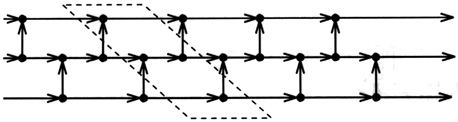Figure 6: Network of transmission lines.

If the network can be split in term of q-junctions, serial, parallel connections of oriented quad-ups and junctions without an oriented loop, then it is simulable, and is also a quad-up.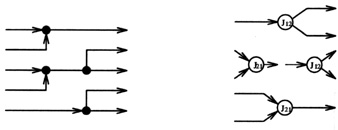Figure 7: Network decomposition.

Fig. 7.a demonstrates how the network may be split into a regular pattern. Figure 7.b represents the pattern (a) as the parallel connection of different quad-ups. Thus this network is simulated by using simple basic elements (only J12 and J21 junctions). Of course this decomposition is not unique, and we could propose other combinations for this network.

## SSM Builders

We developed a statespace library for Maple.55ex©.17em which performs all kinds of symbolic computing. The statespace library implements the three basic combinations known here as augment, connect and feedback. It also implements basic combinations for quadripoles known here as q-junctions and q-append. It also provides facilities for transforming the Maple representation into any other kind of representation. The library computes a transfer function representation from any linear system. It communicates with the control toolbox of Matlab.55ex©.17em. And finally it generates C code which simulates the linear system. The C code may be associated with other pieces of code for simulating non-linear systems.

The Tube library is a library built around the statespace library which implements known linear models: cylindrical tubes with or without viscothermal losses, boundary conditions, junctions and T-junctions.

## SSS builders

The formalism described in the chapter "Modular Representation" can easily be translated into an object-oriented language, such as C++. We've build a C++ library which implements a rather complex inheritance tree around the Module abstract class. Any Module has two primary methods which approximately correspond to the f and g functions (eq. 1.a), and another method for updating its parameters. As shown in figure 8, we derive several different abstract Module subclasses such as Quadripole, Quad_up, Quad_down, Filter...

Usable objects are either Compound Modules, or any non-abstract class which implements a particular system. At this point we use parallel and serial connections, and q-junctions. We also implement classes which represent boundary conditions, tube models with or without viscothermal losses [Mat95], waveguide junctions [Tas94], non-linear reed models [Rod94]. This list may be completed by the implementation of other physical models.

Each non-abstract Module is described by an algorithm which computes an output from the inputs and its internal state. Some internal parameters (such as the reflection coefficient for a boundary condition) can be updated at run-time by a specific method. Others cannot (such as the length of a tube which cannot change dynamically).

We provide users with interactive interface with links, modules and vectors for designing and simulating systems and for controlling the synthesis with time-varying parameters.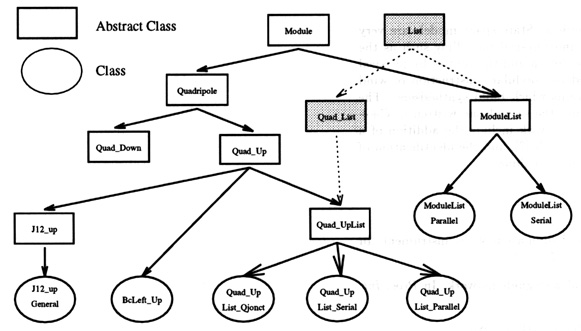Figure 8: Inheritance tree for the Module class.

## Conclusion

We have considered here sound synthesis using state space models. State space models are very promising since they keep the positive aspects of both signal and physical modelling such as the ability for automatic extraction of parameters, the internal description and the control by physical parameters. We described the methodology that we developed for modular construction of wind instruments and software realizations of formal calculus programs which build synthesisers. The first one written in Maple is devoted to theoretical purposes and the second one written in C++ is devoted to sound synthesis. Future development plans for this work include the addition of a fractional delay module in order to simulate tubes of arbitrary length [
Val94], and the identification of unknown physical parameters through the use of Kalman filtering techniques.

### References

DM92
X. Rodet D. Matignon, Ph. Depalle. State space models for wind-instrument synthesis. In Proc. Int. Computer Music Conf. (ICMC'92), pages 142-145, oct. 14-18 1992.

FC90
J. L. Florens and C. Cadoz. Modular modelisation and simulation of the instrument. In Proc. Int. Computer Music Conf. (ICMC'90), pages 75-78, 1990.

GMR88
G. E. Garnett and B. M. Mont-Reynaud. Hierarchical waveguide network. In Proc. Int. Computer Music Conf. (ICMC'88), 1988.

Kai80
T. Kailath. Linear systems. system sciences series. Prentice-Hall, 1980.

Mat90
D. Matignon. Étude de l'application des modèles en variables d'état à l'Analyse-Synthèse. Rapport de stage d'option scientifique à l'IRCAM, École Polytechnique, 1990.

Mat94
D. Matignon. Représentations en variables d'état de modèles de guides d'ondes avec dérivation fractionnaire. PhD thesis, Université Paris XI Orsay, November 1994.

Mat95
D. Matignon. Physical modelling of musical instruments: analysis-synthesis by means of state space representations. In Proc. Int. Symp. of Musical Acoustics (ISMA'95), 1995.

Rod94
X. Rodet. Stability/instability of periodic solutions and chaos in physical models of musical instruments. In Proc. Int. Computer Music Conf. (ICMC'94), pages 386-393, Sept. 12-17 1994.

Smi92
J. Smith. Physical modeling of musical instruments, part i. Computer Music J., 16(4):74-91, Winter 1992.

Tas94
S. Tassart. Génération de modèles en variables d'état par calcul formel. rapport de DEA Acoustique, Traitement du Signal et Informatique appliqué à la Musique, June 1994.

Val94
V. Valimäki. Fractionnal Delay Waveguide Modeling of Acoustic Tubes. Report 34, Helsinsky University of Technology, Lab. of Acoustics and Audio Signal Processing, Espoo, Finland, July 1994.

### Notes

1 A Parralel q-junction is defined as- = (-1,-2),+ = (+1,+2),+ = (+1,+2), and- = (-1,-2).
2 There is no sense in defining a q-feedback for quadripoles.

____________________________
Server © IRCAM-CGP, 1996-2008 - file updated on .

____________________________
Serveur © IRCAM-CGP, 1996-2008 - document mis à jour le .